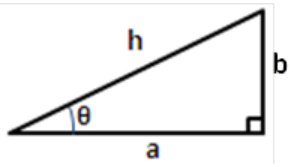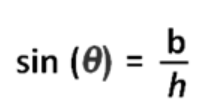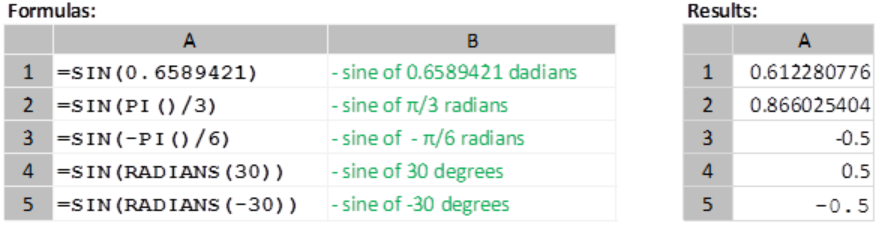Get instant live expert help with Excel or Google Sheets“My Excelchat expert helped me in less than 20 minutes, saving me what would have been 5 hours of work!”

#### Post your problem and you'll get expert help in seconds

Your message must be at least 40 characters
Our professional experts are available now. Your privacy is guaranteed.

# How to Find the Sine of an Angle Using the Excel SIN Function

The Excel SIN function calculates the sine of a given angle. The syntax is as follows:

`SIN(value)`

The value argument of this function is the angle —expressed in radians— of which we want to compute for the sine.

## Sine of an Angle

As a refresher to the concept of sine, consider the right triangle below. For this simple right-angled triangle, the sine of the angle θ, is the ratio of the opposite side, b, to the hypotenuse, h.Figure 1. Find the Sine of an Angle Using SIN Function

Therefore, for the triangle shown above,

##Figure 2. Find the Sine of an Angle Using SIN Function

## Converting Angles from Degrees to Radians

If the angle we are computing the sine for is in degrees, we will need to convert it into radians before using it as an argument to the SIN function. Remember that the SIN function expects radians. This is where we use another very useful Excel function: RADIANS. The syntax of the RADIANS function is as follows:

`RADIANS(degrees)`

## Examples of Using the SIN Function

Consider the following examples:Figure 3. Find the Sine of an Angle Using SIN Function

In the examples shown in Fig.1, note that

• The Excel PI function is used to supply the values π/6 and -π/3 respectively in cells A2 and A3. The PI function returns the value of pi (π).
• In cells A4 and A5, the RADIANS function is used to convert the angles of 30 degrees and -30 degrees to radians before they are supplied as arguments for the SIN function.

### Did this post not answer your question? Get a solution from connecting with the expert.Another blog reader asked this question today on Excelchat:## Subscribe to Excelchat.coAnother blog reader asked this question today on Excelchat: